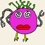### Adding an isolated 1-chain. Dots and Boxes

1 replies. Last post: 2007-05-19

•wccanard at 2007-05-19

In trying to be Berlekamp's Most Assiduous Reader,

and in particular trying to fill in the details of

some of the assertions he makes in chapter 12, I've

been led to trying to understand how the value of a game

can change if you add an isolated chain of length 1.

Let v(G) be the value (to the second player) of the game G

and let G+1 denote G plus the isolated chain. What is

immediately clear is that v(G)+v(G+1) is less than or equal to 1, because one strategy for the first player in G+1 is to open the 1-chain. Equality can certainly be attained—for example if G is an isolated long chain then v(G)+v(G+1)=1.

At the other extreme, if G has nim-value 2 and a very long chain then v(G) and v(G+1) will both be highly negative because the first player takes the nim-value down to zero and claims the long chain, in both cases. By trying some simple positions with nim-value 2 one easily sees that one can find a game G such that v(G) and v(G+1) are negative and that \|v(G)-v(G+1)\|=1. One can also get \|v(G)-v(G+1)\|=3 this way. Note that the difference is certainly odd because the number of boxes changes by 1.

Can v(G) and v(G+1) ever both be negative and differ in absolute value by 5? By 7? By more? Oh—the answer is “yes” if you allow loony positions so let me furthermore restrict to the case where G contains no capturable coins (thus ruling out all loony positions and more besides).

Can one even write down the complete set of possibilities for the unordered pair {v(G),v(G+1)}?

Note also that v(G)=v(G+1+1) by a man-in-the-middle argument.

wc